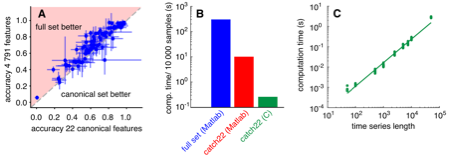## Tuesday, 1 April 2014

### Fast inference about noisy biology

Biology is a random and noisy world -- as we've written about several times before! (e.g. here and here) This often means that when we try to measure something in biology -- for example, the number of a particular type of proteins in a cell, or the size of a cell -- we'll get rather different results in each cell we look at, because random differences between cells mean that the exact numbers are different in each case. How can we find a "true" picture? This is rather like working out if a coin is biased by looking at lots of coin-flip results.

Measuring these random differences between cells can actually tell us more about the underlying mechanisms for things like (to use the examples above) the cellular population of proteins, or cellular growth. However, it's not always straightforward to see how to use these measurements to fill out the details in models of these mechanisms. A model of a biological process (or any other process in the world) may have several "parameters" -- important numbers which determine how the model behaves (the bias of a coin, is an example, telling us what proportion of times we'll see heads). These parameters may include, for example, rates with which proteins are produced and degraded. The task of using measurements to determine the values of these parameters in a model is generally called "parametric inference". In a new paper, I describe a new and efficient way of performing this parametric inference given measurements of the mean and variance of biological quantities. This allows us to find a suitable model for a system describing both the average behaviour and typical departures from this average: the amount of randomness in the system. The algorithm I propose is an example of approximate Bayesian computation (ABC) which allows us to deal with rather "messy" data: I also describe a fast (analytic) approach that can be used when the data is less messy (Normally distributed).Parametric inference often consists of picking a trial set of parameters for a model and seeing if the model with those parameters does a good job of matching experimental data. If so, those parameters are recorded as a "good" set, otherwise, they're discarded as a "bad" set. The increase in efficiency in my proposed approach is due to the fact that we can perform a quick, preliminary check to see if a particular parameterisation is "bad", before spending more computer time on rigorously showing that it is "good". I show a couple of examples in which this preliminary checking (based on fast computation of mean results before using stochastic simulation to compute variances) speeds up the process by 20-50% on model biological problems -- hopefully allowing some scientists to grab a little more coffee time! This work will be coming out in the journal Statistical Applications in Genetics and Molecular Biology with the title `Efficient parametric inference for stochastic biological systems with measured variability' and you'll find the article (free) here. Iain